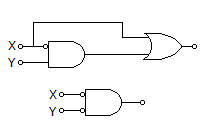# Electronics and Communication Engineering - Digital Electronics

36.

The logic operations of the two circuits given below areA. complementary B. identical C. entirely different D. none of these

Answer: Option C

Explanation:

No answer description available for this question. Let us discuss.

37.

Which of the following is incorrect?

 A. (111)2 + (10)2 = 9 B. (111)2 + (2)10 = (9)16 C. 7 + 2 = (1001)2 D. None of the above

Answer: Option D

Explanation:

No answer description available for this question. Let us discuss.

38.

A register is

 A. a group is memories B. a group of devices that store digital data C. a chip used in computers D. a pure silica piece used in digital systems

Answer: Option B

Explanation:

No answer description available for this question. Let us discuss.

39.

In 8085 microprocessor, when TRAP interrupt is triggered program control is transferred to location

 A. 0020 H B. 0024 H C. 0028 H D. 002 CH

Answer: Option B

Explanation:

No answer description available for this question. Let us discuss.

40.

In 8085 microprocessor, what is the length of SP (stack) pointer?

 A. 6 bits B. 8 bits C. 12 bits D. 16 bits

Answer: Option D

Explanation:

No answer description available for this question. Let us discuss.

#### Current Affairs 2021

Interview Questions and Answers